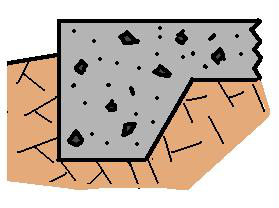* Log In to use the Calculate function * Become a Member!

###### Design of Concrete Floors-on-Grade:(Reference: Unified Facility Design Criteria - Concrete Floor Slabs on Grade Subjected to Heavy Loads)

Floors - Max. Stationary LL
• Calculate the maximum stationary live load that concrete slab-on-grade floor can support.

Floors - Thickness For Sta. LL
• Calculate the minimum thickness required for a concrete slab-on-grade floor to support a stationary live load.
• Calculate the required concrete slab-on-grade floor thickness required to support traffic loads.
Floors on Modified Soil
• Calculate the required floor thickness for a concrete slab-on-grade floor supported by a stabilized/modified soil subgrade.
Floors - Reinforced Slab Length
• Calculate the maximum length of steel reinforced slab-on-grade floors.
Floors - Required Reinforcement
• Calculate the minimum steel reinforcement required to reduce the required slab-on-grade concrete thickness by two or three inches.
Concrete - Flexural Strength
• Calculate the approximate flexural strength of concrete (S'c) from the 28 day compressive strength (f'c).
Concrete - Compressive Strength
• Calculate the approximate 28 day compressive strength of concrete (f'c) from the 28 day flexural strength (S'c).
California Bearing Ratio, CBR
• Calculate the California Bearing Ratio, CBR, from the Modulus of Soil Reaction, k.
Modulus of Soil Reaction, k
• Calculate the Modulus of Soil Reaction, k from the California Bearing Ratio, CBR.
Floors - Max. Edge Wall Load
• Calculate the maximum wall load that the edge of a concrete slab-on-grade floor can support.
Floors - Edge Wall Ld Thickness
• Calculate the thickness of the edge of a concrete slab-on-grade floor required to support a wall load.
• Calculate the maximum wall load that the maximum wall load that a concrete slab-on-grade floor can support at a joint or at the center/interior of the slab.
Floors - Ctr Wall Ld Thickness
• Calculate the required thickness of a concrete slab-on-grade floor to support a wall load at the center/interior or on a joint.
Floors - Fiber Reinforcement
• Calculate the minimum thickness of a fiber reinforced slab-on-grade floor to support traffic loads.
Floors - Fiber Deflection